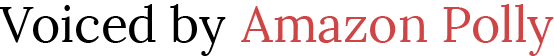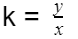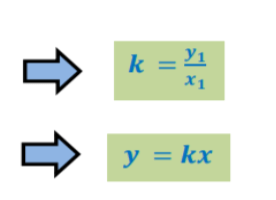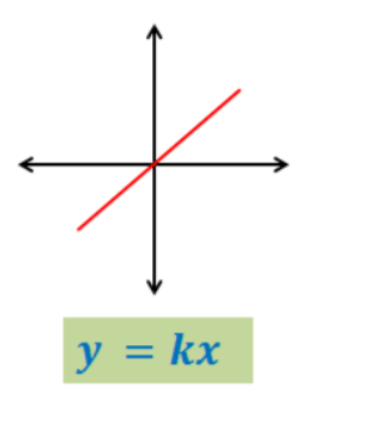# Teaching Direct Variation

 Would You Rather Listen to the Lesson?In units on linear functions, students learn about direct variation. This includes learning what the concept of direct variation in relations means, learning how to represent a direct variation using an equation, as well as graphing directly varying relations.

To make lessons on direct variation more accessible and engaging, math teachers can employ a variety of teaching strategies. In this article, we bring you a few such strategies. Use them in your class, and never worry about teaching direct variation again!## Strategies to Teach Direct Variation

### What Is Direct Variation?

You can start your lesson by providing a definition of direct variation. Explain that direct variation is a relationship between two quantities that can be represented by a function of the following form:

y = kx

…where k does not equal 0. Add that k is the coefficient of x, and x and y vary directly with each other. Point out we can find the value of k as:

####Example 1:

Write an equation on the whiteboard and ask students if it represents a direct variation. Then, demonstrate how we can find the constant of variation or k. For example, you can write the following equation:

5y = -3x

Point out that we can rewrite the equation in the form of y = kx, which will result in:From the above, we can infer that the constant of variation is as follows:

####Example 2:

Write another equation on the whiteboard and ask students if it’s a direct variation. Ask students to show how we can find the constant of variation k. For instance, you can write the following equation:

3x + 4y = 6

Point out that we’ll add – 3x on both sides and then divide both sides by 4 to simplify:Add that a simple way to know whether an equation represents a direct variation is by checking if there are any lone integers. If there are such integers, then the equation does not represent a direct variation and vice versa.

### Writing Equations of Direct Variations

Explain to students how we can write the equation of a direct variation. Point out that if we are given any ordered pair (𝑥1,𝑦1) for a relationship that has a direct variation, we will find the constant of variation k and then write the equation, that is:#### Example:

Provide an example of how we can write an equation of a direct variation. For instance, suppose y varies directly with x and y = 21 when x = 3. Demonstrate to students how we can write a direct variation equation relating x and y.

Point out that by applying what we already know, we will first find the constant of variation k. We know that y equals 21 and x equals 3, so we will simply plug them in the mentioned formula and thus find k:Add that now we can simply write the equation:

y = kx

y = 7x

### Graphing a Direct Variation

Point out to students that the graph of a direct variation is a straight line that passes through the origin (0,0). Present an example so that students can visualize what the graph will look like. For instance, on the image below, we can observe the graph of direct variation:You can also enrich your lesson on direct variation with multimedia material, such as videos. For instance, use this video by Khan Academy to introduce the concept of direct variation, as well as illustrate it with examples.

This video is also a good resource to present simple guidelines on how to check whether an equation is a direct variation, as well as how to write the equation of a direct variation. Finally, use this video to show how to graph a direct variation.## Activities to Practice Direct Variation

### Online Jeopardy Game

This is an online game that will help students improve their skills to write a direct variation equation given x and y, and identify whether a given equation is a direct variation or find the constant of variation. Make sure that all students have a suitable device before starting.

Divide students into groups of three. Each person in the group represents a team of their own and competes against the other two players. Students should select the three-teams mode in the game when starting to play.

Players in each group take turns to answer questions related to direct variation. Each player selects the category of questions and the difficulty level. Depending on the difficulty of the question, they can score from 100 to 500 points if they answer correctly.

In the end, the student with the highest score is declared a winner. Parents who are homeschooling their children can also adjust the game to an individual one, by choosing the one-team mode when starting the game.

### Group Work

This game will help students hone their skills to determine if an equation is a direct variation, as well as show them how to represent a direct variation using an equation. To use this activity in your class, print out this Assignment Worksheet (Members Only).

Divide students into groups of 3 or 4 and hand out the worksheets (one worksheet per student). Provide instructions. Explain that students in each group work together to answer the question in the worksheet.

Provide some 15 minutes for students to complete the exercises. In the end, provide space for discussion and reflection. Which strategies did students use to determine if an equation is a direct variation? Were there any exercises that were particularly challenging? Why?

Before You Leave…

If you liked the strategies on teaching direct variation that we outlined in this article, we have a whole lesson that is dedicated to this topic!

So if you’re looking for more math materials for children of all ages, sign up for our emails for plenty of free content! Feel free to also head over to our blog, and check out our free resources on teaching functions!

And if you’d like to become a member, sign up at Math Teacher Coach!